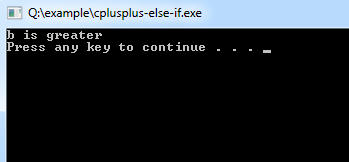## C++ Programming Tutorial Index

C++ `else-if` is like another `if` condition; It is useful when there are possibilities of multiple `if` and based on that, some statements need to be executed. This tutorial will teach you how to use `else-if` Statements in C++.

The basic format of the `else-if` Statement is:

Syntax:

``````if(test_expression)
{
}
else if(test_expression n)
{
}
else
{
}``````

Example of a C++ program to demonstrate `else-if` Statement:

Example:

``````#include <iostream>
using namespace std;

int main()
{
int a=15,b=20;

if(b>a)
{
cout << "b is greater"<<endl;
}else if(a>b){
cout << "a is greater"<<endl;
}
else
{
cout << "\n Both are equal"<<endl;
}
system("PAUSE");
}``````

Program Output: